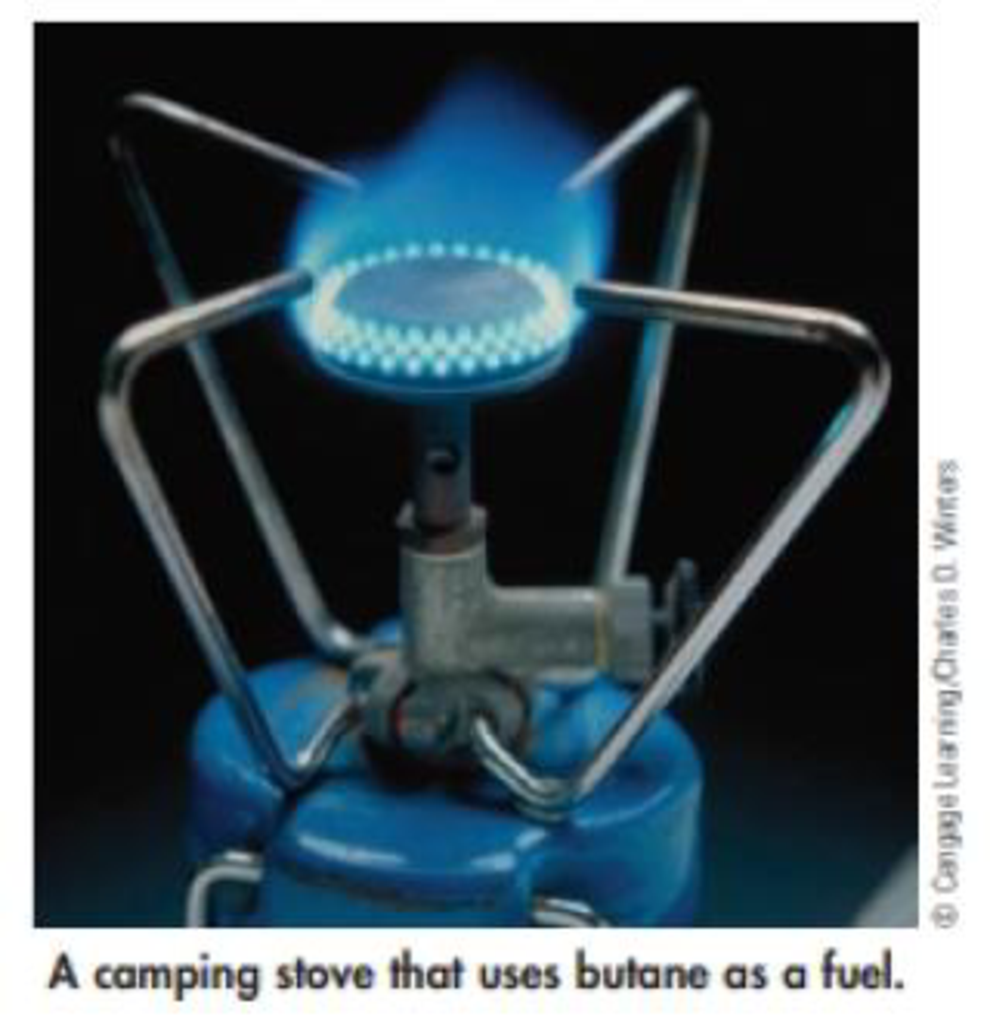# Camping stoves are fueled by propane (C 3 H 8 ), butane [C 4 H 10 (g), Δ f H ° = –127.1 kJ/mol]. gasoline. or ethanol (C 2 H 5 OH). Calculate the enthalpy of combustion per gram of each of these fuels. [Assume that gasoline is represented by isooctane, C 8 H 18 ( ℓ ) , with Δ f H ° = –259.3 kJ/mol.) Do you notice any great differences among these fuels? How are these differences related to their composition?### Chemistry & Chemical Reactivity

9th Edition
John C. Kotz + 3 others
Publisher: Cengage Learning
ISBN: 9781133949640

#### Solutions

Chapter
Section### Chemistry & Chemical Reactivity

9th Edition
John C. Kotz + 3 others
Publisher: Cengage Learning
ISBN: 9781133949640
Chapter 5, Problem 84GQ
Textbook Problem
43 views

## Camping stoves are fueled by propane (C3H8), butane [C4H10(g), ΔfH° = –127.1 kJ/mol]. gasoline. or ethanol (C2H5OH). Calculate the enthalpy of combustion per gram of each of these fuels. [Assume that gasoline is represented by isooctane, C8H18(ℓ), with ΔfH° = –259.3 kJ/mol.) Do you notice any great differences among these fuels? How are these differences related to their composition?Interpretation Introduction

Interpretation:

The enthalpy of combustion per gram of propane and butane has to be calculated

Concept Introduction:

The standard enthalpy change of combustion of a compound is the enthalpy change which occurs when one gram of the compound is burned completely in oxygen under standard conditions, and with everything in its standard state

ΔrH0=ΣnΔfH0(products)-ΣnΔfH0(reactants)

The change in enthalpy, ΔH in kJ per mole of a given reactant for the reaction can be calculated as:

ΔrH=enthalpy changenumber of moles

ΔH=ΔrH×number of moles

### Explanation of Solution

For propane the balanced equation is as follows:

C3H8+ 5 O23CO2+ 4 H2O

ΔrH0=[(3mol×-393.5kJ/mol)+(4mol×-285.83kJ/mol)]-[(1mol×-104.7 kJ/mol+0)]

ΔrH0=-2219.12kJ

The change in enthalpy, ΔH in kJ per mole of a given reactant for the reaction can be calculated as:

ΔrH=enthalpy changenumber of moles

ΔrH=-2219.12kJ/mol×1mol44.1g=-50.32J/g.K

So, the enthalpy of combustion is -50.32J/g

### Still sussing out bartleby?

Check out a sample textbook solution.

See a sample solution

#### The Solution to Your Study Problems

Bartleby provides explanations to thousands of textbook problems written by our experts, many with advanced degrees!

Get Started

Find more solutions based on key concepts
Cells a. are self-contained, living units. b. serve the bodys needs but have few needs of their own. c. remain ...

Nutrition: Concepts and Controversies - Standalone book (MindTap Course List)

How does the presence of degenerate matter in a star trigger the helium flash?

Horizons: Exploring the Universe (MindTap Course List)

31-8 What are memory cells? What is their function?

Introduction to General, Organic and Biochemistry

Name two strategies for increasing a school-age childs physical activity.

Nutrition Through the Life Cycle (MindTap Course List)

(a) Explain how the quality factor is related to the response characteristics of a radio receiver. (b) Which va...

Physics for Scientists and Engineers, Technology Update (No access codes included)

In a pressure cooker, water boils at 120C. What is the pressure inside the cooker?

Physics for Scientists and Engineers: Foundations and Connections

Why do you think the mid-ocean ridges are higher than the surrounding seabed?

Oceanography: An Invitation To Marine Science, Loose-leaf Versin